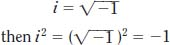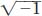NextPrevious

# What are imaginary numbers?

The “opposite” of real numbers are (logically enough) called imaginary numbers. In particular, they are all non-zero multiples (real numbers) of the square root of -1, also called i, with the formula defined as follows:Thedoes not have a position on a number line; and no number can be squared to get -1. (If you square a positive number, the result is positive; if you square a negative number, the result is also a positive number.) Thus, in order to square a number to get a negative one, mathematicians invented the imaginary number i.

Close

This is a web preview of the "The Handy Math Answer Book" app. Many features only work on your mobile device. If you like what you see, we hope you will consider buying. Get the App